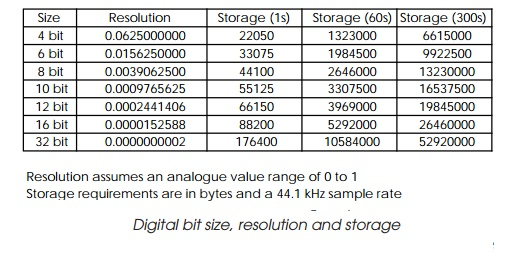Home | | Embedded Systems Design | Analogue to digital conversion techniques

# Analogue to digital conversion techniques

The basic principle behind analogue to digital conversion is simple and straightforward: the analogue signal is sampled at a regular interval and each sample is divided or quantised by a given value to determine the number of given units of value that approximate to the analogue value.

Analogue to digital conversion techniques

The basic principle behind analogue to digital conversion is simple and straightforward: the analogue signal is sampled at a regular interval and each sample is divided or quantised by a given value to determine the number of given units of value that approximate to the analogue value. This number is the digital equivalent of the analogue signal.The combination graph shows the general principle. The grey curve represents an analogue signal which, in this case, is a sine wave. For each cycle of the sine wave, 13 digital samples are taken which encode the digital representation of the signal.

Quantisation errors

Careful examination of the combination chart reveals that all is not well. Note the samples at time points 7 and 13. These should be zero â€” however, the conversion process does not convert them to zero but to a slightly higher value. The other points show similar errors; this is the first type of error that the conversion process can cause. These errors are known as quantisation errors and are caused by the fact that the digital representation is step based and consists of a selection from one of a fixed number of values. The analogue signal has an infinite range of values and the difference between the digital value the conver-sion process has selected and the analogue value is the quantisation error.The size of the quantisation error is dependent on the number of bits used to represent the analogue value. The table shows the resolution that can be achieved for various digital sizes. As the table depicts, the larger the digital representation, the finer the analogue resolution and therefore the smaller the quantisation error and resultant distortion. However, the table also shows the increase which occurs with the amount of storage needed. It assumes a sample rate of 44.1 kHz, which is the same rate as used with an audio CD. To store five minutes of 16 bit audio would take about 26 Mbytes of storage. For a stereo signal, it would be twice this value.

Study Material, Lecturing Notes, Assignment, Reference, Wiki description explanation, brief detail
Embedded Systems Design : Interfacing to the analogue world : Analogue to digital conversion techniques |